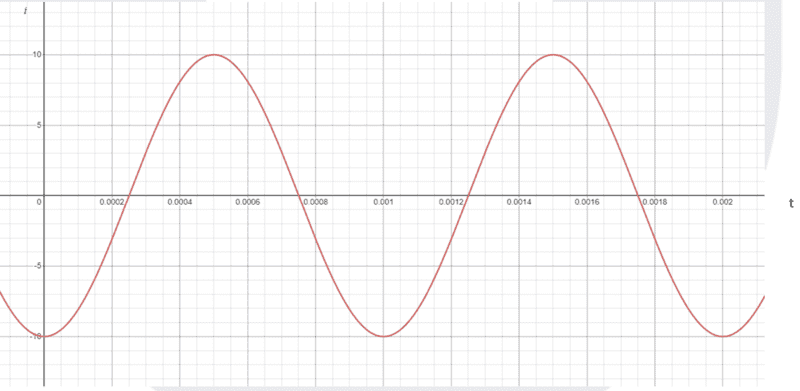# Rearrange formula and work out phase angle

• leejohnson222
In summary, the conversation discussed the values of amplitude and frequency, as well as obtaining the phase angle for a sinusoidal wave. It was mentioned that the phase angle can be obtained by plugging in a value for t, reading off the y value from the graph, and solving the equation. The ambiguity in evaluating ##\arcsin## was also addressed, and it was suggested to use two points for more accuracy. Finally, there was a disagreement about the correct method for obtaining the phase angle.

#### leejohnson222

Homework Statement
work out what ip, f and phase angle is, then rearrange the formula to make t the subject and find out at what point in time does 6.78 amp occur
i = Ipsin (2pi f t - phase angle)

so far i think Ip = 10 f = 1000hz
struggling with the phase angle
Relevant Equations
i = Ipsin (2pi f t - phase angle)
so far i think Ip = 10 f = 1000hz
struggling with the phase angleI confirm your amplitude and frequency values.
To get the phase angle, plug in a value for t, read off the y value from the graph and solve the equation. You will need to do that for at least two points because of the ambiguity in evaluating ##\arcsin##.

Shouldn't the amplitude have units?
haruspex said:
To get the phase angle, plug in a value for t, read off the y value from the graph and solve the equation. You will need to do that for at least two points because of the ambiguity in evaluating ##\arcsin##.
Can the phase angle not be obtained by noting that at t = 0 the sinusoidal is at a minimum?

kuruman said:
Shouldn't the amplitude have units?

Can the phase angle not be obtained by noting that at t = 0 the sinusoidal is at a minimum?
I should have written "you may have to". I was trying to be general.

i could be very wrong here, but i worked out the angle to be 90 degrees ?
i full cycle of the wave is 0.001 s and the delay from 0 is 0.00025 s
360 x 0.00025 / 0.001 = 90

That's not what @haruspex suggested ... but I agree.

Easy:
##I = I_p\sin (2\pi f t - \phi)\quad ## pick ##t=0## and solve ##\sin(-\phi)=-1\ ##

Personally, I prefer to imagine the graph for ##\phi = 0## and conclude that a shift of ##-{\pi\over 4}## to the right yields the given graph. Therefore ##\ -\phi=-{\pi\over 4}##

##\ ##